# Methods and formulas for T² Chart

Select the method or formula of your choice.

## Plotted points

### Data in subgroups

When data are in subgroups, T2 is calculated as follows:

where: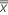is the mean vector of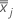(mean of xjk values), which is calculated as follows:

S = sample covariance matrix

The sample covariance matrix, S, is calculated as follows:

where:

where: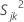, the sample variance for the jth characteristic in the kth sample, is calculated as follows:

where:

where: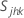, the covariance, =

The average of the S matrices is an unbiased estimate of the variance when the process is in control. n must be greater than p and there must be no strong correlations between the variables so that the sample covariance matrix is not singular.

### Individual observations

When data are individual observations, T2 is calculated as follows:

where:

where:

### Notation

TermDescription
nsample sizesample mean vector
xijkthe ith observation on the jth characteristic in the kth sample
mnumber of samples

## Example of calculating T2

Minitab plots the T2 statistic on a control chart. If a plotted point exceeds the control limits, the process is out of control at that point. See the table and sample equations for reference on Minitab calculations.

The following data come from a cleaning solution development process. The amounts of sodium citrate and glycerine affect the potency of the solution.

 Subgroup Means Variances and Covariances T2 Statistic Subgroup Sodium citrate (X1) Glycerin (X2) S 1 2 S2 2 S 1 2 k T2 1 125 025 7292 8692 5791 5708 2 625 4 2292 2333 3333 1429 3 4 875 1467 0625 8000 9528 4 2 2 2933 7600 6667 8073 5 25 225 2500 2692 7917 7548 6 4 45 6667 9567 3333 2711 7 275 025 3692 4692 7108 7785 8 6 65 4333 7700 6933 6183 9 625 325 7892 5558 1325 3592 10 3 5 2867 9467 2600 4942 11 25 5 1767 1200 9000 3279 12 1 625 1467 1692 4033 0277 Averages 7875 2333 7931 9318 3003
1. Calculate the subgroup means for each variable, X1 and X2. In this case, each subgroup had four samples.
2. If you have individual observations, Minitab uses them instead of the subgroup means in all calculations.
3. Calculate the subgroup variances, S1 2 and S2 2.
4. Calculate the subgroup covariances, S1 2 k.
5. Calculate the means of the subgroup means, the means of subgroup variances, and the mean of the covariances.
6. Designate the sample covariance matrix S and the mean vector.
7. Calculate T2, which is given by:

Minitab plots T2 on the T2 chart and compares it to the control limits to determine if individual points are out of control.

## Center line

The center line for the T2 chart is KX. The calculation of K and X depends on the maximum sample size and whether Minitab estimates the covariance matrix from the data.

### Data in subgroups

When data are in subgroups, KX is calculated as follows:

Given covariance matrix
Estimated covariance matrix

### Individual observations

When data are individual observations, KX is calculated as follows:

Given covariance matrix
Estimated covariance matrix

where:

### Notation

TermDescription
Pnumber of variables
Mnumber of subgroups
Nsample size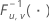the inverse cumulative F distribution with u numerator degrees of freedom and v denominator degrees of freedom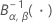the inverse cumulative beta distribution with first shape parameter α and second shape parameter β

## Control limit

### Data in subgroups

The upper control limit if you do not specify parameters is:

The upper control limit if you specify parameters is:

### Individual observations

The upper control limit if you do not specify parameters is:

where:

See Woodall et al.1 for more information.

The upper control limit if you specify parameters is:

### Notation

TermDescription
αfixed value of 0.00134989803156746
pnumber of characteristics
m

For data in subgroups, if you do not specify parameter estimates, then m is the number of samples. If you do provide parameter estimates, then m is the number of samples used to create the covariance matrix.

For individuals data, m is the number of observations.

nsize of each sample
Findicates that the F distribution is used
Bindicates that the beta distribution is used

## Decomposed T2 statistic

Decomposed T2 statistic:

where:

where:

xi(p − 1)is the decomposed mean vector

Sxx is the (p – 1) × (p – 1) principal submatrix of S

T2p|1,..., p−1 is an approximation that differs for phases and whether you have subgroups or individual observations:

Phase 1 for data in subgroups:

Phase 2 for data in subgroups:

Phase 1 for individual observations:

Phase 2 for individual observations:

Minitab calculates phase 1 control limits when you don't specify parameter estimates and phase 2 control limits when you do.

See Mason et al.2 for more information on the Decomposed T2 statistic.

### Notation

TermDescription
mnumber of samples
Findicates that the F distribution is used
Bindicates that the beta distribution is used

## Methods and formulas for Box-Cox

### Box-Cox formula

If you use a Box-Cox transformation, Minitab transforms the original data values (Yi) according to the following formula:

where λ is the parameter for the transformation. Minitab then creates a control chart of the transformed data values (Wi). To learn how Minitab chooses the optimal value for λ, go to Methods and formulas for Box-Cox Transformation.

### Common λ values

The following table shows some commonly used λ values and their transformations.
λ Transformation
2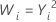0.5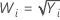0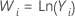−0.5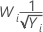−1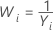1 J. H. Woodall and W.H. Sullivan (1996). "A Comparison of Multivariate Control Charts for Individual Observations," Journal of Quality Technology, 28 (4), 398-408.
2 R. L. Mason, N. D. Tracy, and J. C. Young. (1995). "Decomposition of T 2 for Multivariate Control Chart Interpretation," Journal of Quality Technology , 27, April, 99-108.
By using this site you agree to the use of cookies for analytics and personalized content.  Read our policy